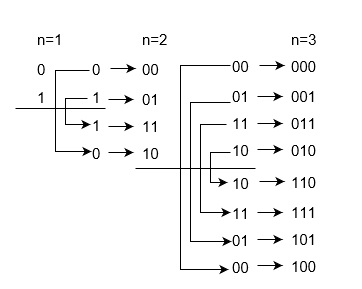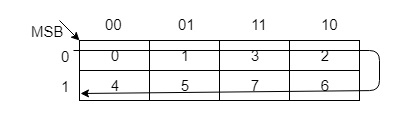# What is Gray code?

The reflected binary code or Gray code is an ordering of the binary numeral system such that two successive values differ in only one bit (binary digit). Gray codes are very useful in the normal sequence of binary numbers generated by the hardware that may cause an error or ambiguity during the transition from one number to the next. So, the Gray code can eliminate this problem easily since only one bit changes its value during any transition between two numbers.

Gray code is not weighted that means it does not depends on positional value of digit. This cyclic variable code that means every transition from one value to the next value involves only one bit change.

Gray code also known as reflected binary code, because the first (n/2) values compare with those of the last (n/2) values, but in reverse order.

## Constructing an n-bit Gray code

n-bit Gray code can be generated recursively using reflect and prefix method which is explained as following below.

• Generate code for n=1: 0 and 1 code.
• Take previous code in sequence: 0 and 1.
• Add reversed codes in the following list: 0, 1, 1 and 0.
• Now add prefix 0 for original previous code and prefix 1 for new generated code: 00, 01, 11, and 10.

Therefore, Gray code 0 and 1 are for Binary number 0 and 1 respectively. Gray codes: 00. 01, 11, and 10 are for Binary numbers: 00, 01, 10, and 11 respectively. Similarly you can construct Gray code for 3 bit binary numbers:Therefore, Gray codes are as following below,

For n = 1 bit
For n = 2 bit
For n = 3 bit
Binary
Gray
Binary
Gray
Binary
Gray
0
0
00
00
000
000
1
1
01
01
001
001

10
11
010
011
11
10
011
010

100
110
101
111
110
101
111
100

Iterative method of generating G(n+1) from Gn are given below. This is simpler method to contract Gray code of n-bit Binary numbers. Each bit is inverted if the next higher bit of the input value is set to one. The nth Gray code is obtained by computing n⊕(floor(n/2)).

• Gn is unique numbers for the permutation from 0  to (2n-1).
• Gn is embedded as the first half of G(n+1) and second half as the reverse order of G(n+1).
• Prefix 0 in each digit of first half and 1 in each digit of second half.

The hamming distance of two neighbours Gray codes is always 1 and also first Gray code and last Gray code also has Hamming distance is always 1, so it is also called Cyclic codes.

You can construct Gray codes using other methods but they may not be performed in parallel like given above method. For example, 3 bit Gray codes can be contracted using K-map which is given as following below:Decimal
Binary
Gray Code
0
000
000
1
001
001
2
010
011
3
011
010
4
100
110
5
101
111
6
110
101
7
111
100

## Types of Gray Codes

There are also other types of Gray codes, like Beckett-Gray code, Single track Gray codes etc.

• N-ary Gray code, where non-Boolean values are included like sequences of 1, 2, 3.
• Two dimensional (n,k) Gray codes are used for error correction.
• Balanced Gray codes has equal transition counts.

## Uses of Gray codes

Gray codes are used in rotary and optical encoders, Karnaugh maps, and error detection.

Updated on: 30-Jun-2020

29K+ Views